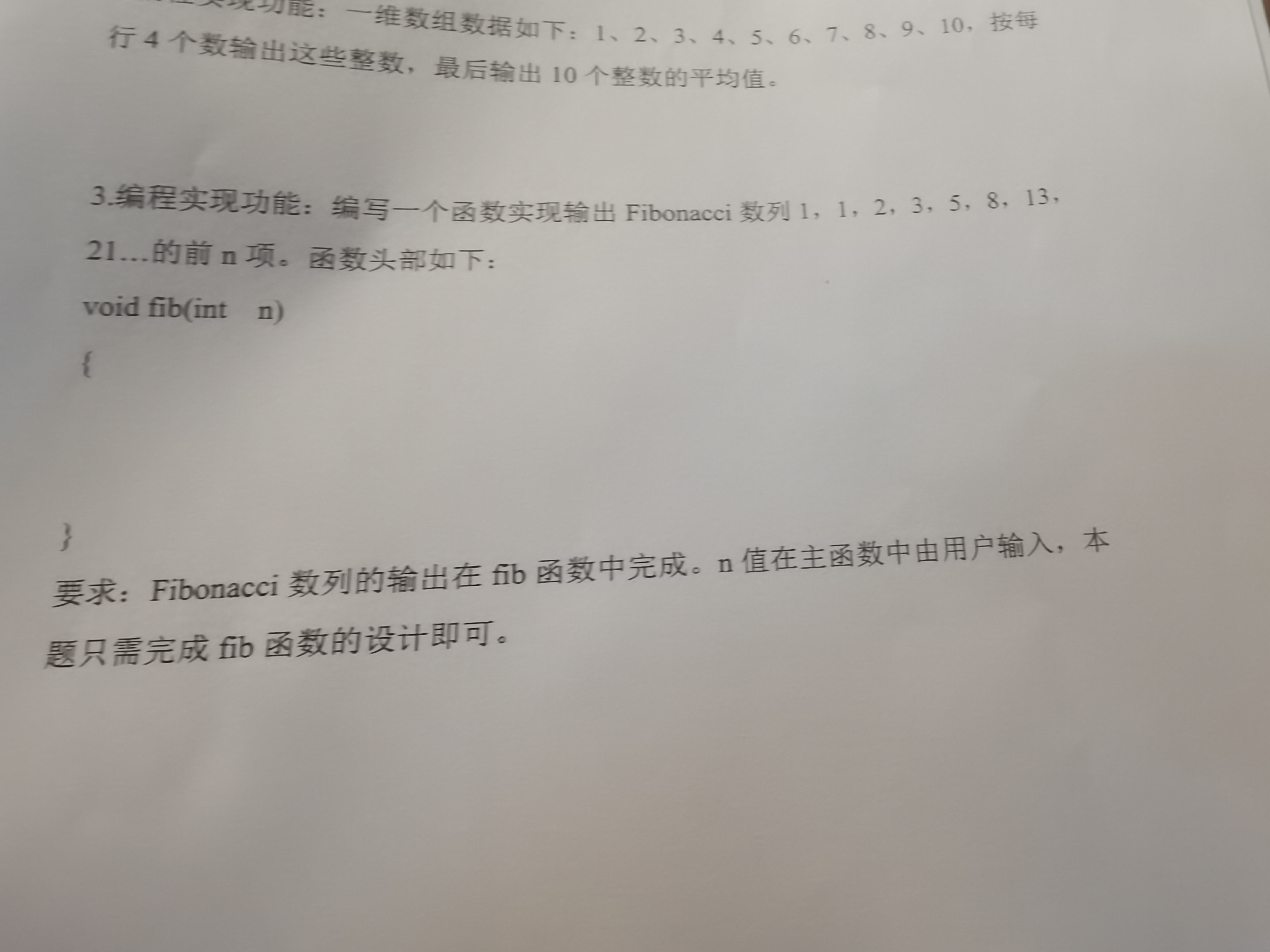2021-06-23 20:15

# C语言中fib函数设计• 写回答
• 好问题 提建议
• 关注问题
• 收藏
• 邀请回答

#### 2条回答默认 最新

•暖晴的天 2021-06-23 20:23
已采纳

代码如下：

``````void fib(int n)
{
int i = 1;
int a;
a = 1;
a = 1;
for (; i <= n; i++)
{
if (i == 1 || i == 2)
{
printf("1 ");
}
else
{
a[i] = a[i - 1] + a[i - 2];
printf("%d ", a[i]);
}
}
}``````
已采纳该答案
评论
解决 无用
打赏 举报
•说明一下定义：斐波那契数列（Fibonacci sequence），又称黄金分割数列，因数学家莱昂纳多·斐波那契（Leonardoda Fibonacci）以兔子繁殖为例子而引入，故又称为“兔子数列”，指的是这样一个数列：0、1、1、2、3、5、8、13、21、34、……在数学上，斐波那契数列以如下被以递推的方法定义：F(0)=0，F(1)=1, F(n)=F(n - 1)+F(n - 2)（n ≥ 2，n ∈ N*）在现代物理、准晶体结构、化学等领域，斐波纳契数列都有直接的应用

``````void fib(int n)
{
int a0 = 0;
int a1 = 1;
int a2;
printf("%d,%d",a0,a1);
for(int i=2;i<n;i++)
{
a2 = a0+a1;
printf(",%d",a2);
a0 = a1;
a1 = a2;
}
}``````
评论
解决 无用
打赏 举报